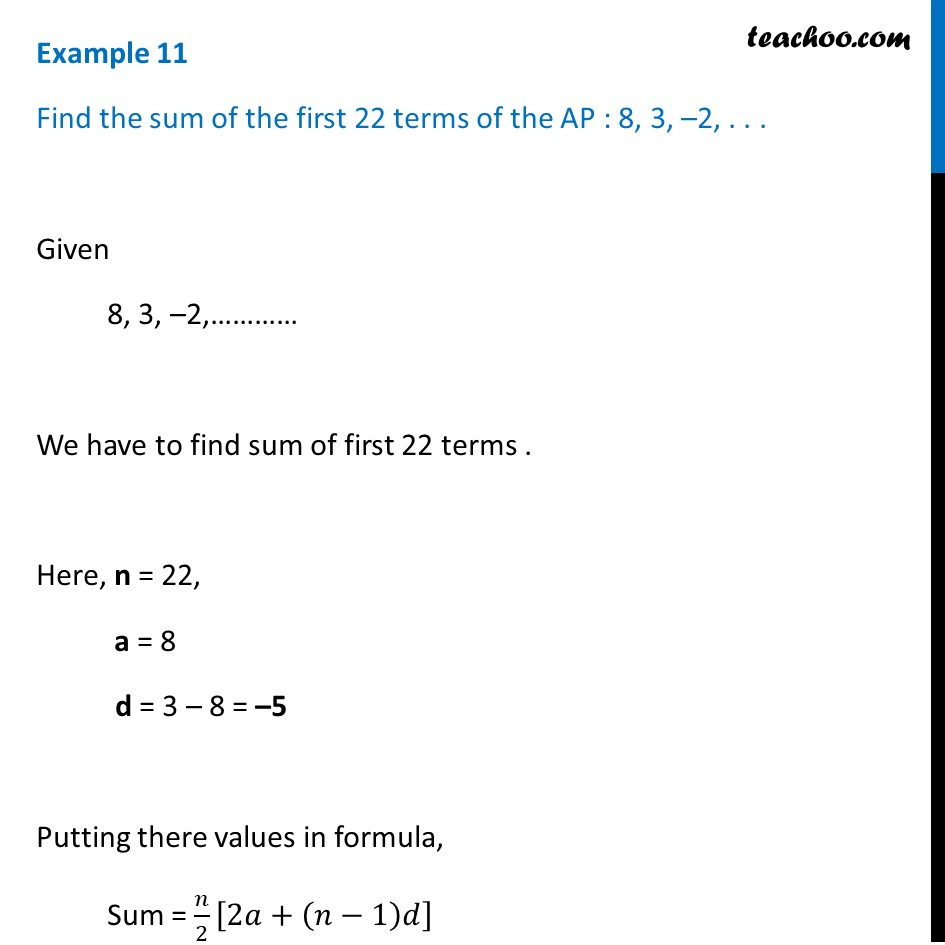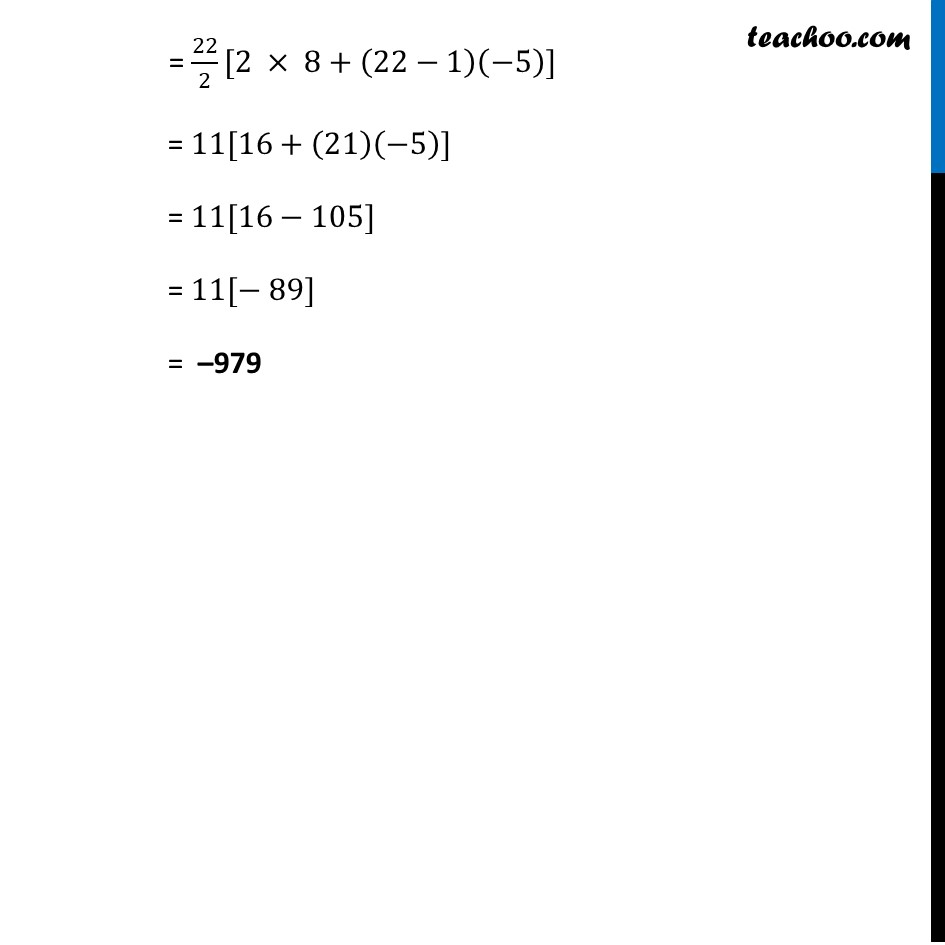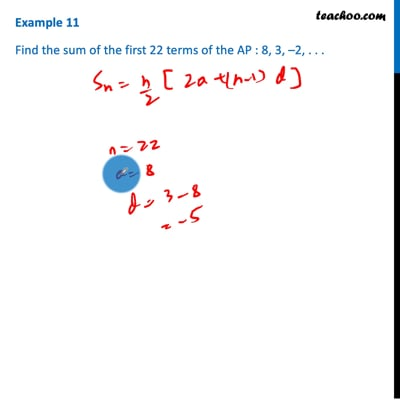Finding sum of n terms

Chapter 5 Class 10 Arithmetic Progressions
Concept wiseThis video is only available for Teachoo black users

Introducing your new favourite teacher - Teachoo Black, at only ₹83 per month

### Transcript

Example 11 Find the sum of the first 22 terms of the AP : 8, 3, –2, . . . Given 8, 3, –2,………… We have to find sum of first 22 terms . Here, n = 22, a = 8 d = 3 – 8 = –5 Putting there values in formula, Sum = 𝑛/2 [2𝑎+(𝑛−1)𝑑] = 22/2[2 × 8+(22−1)(−5)] = 11[16+(21)(−5)] = 11[16−105] = 11[− 89] = –979